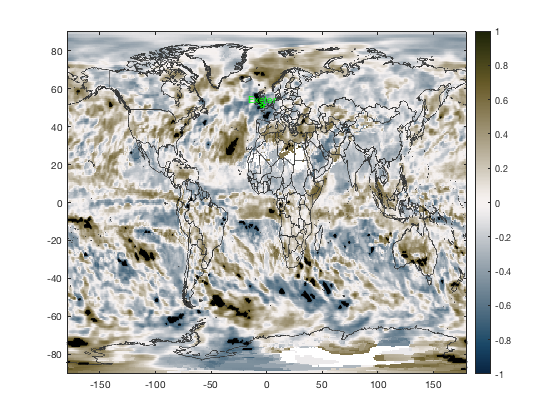# corr3 documentation

corr3 computes linear or rank correlation for a time series and a 3D dataset.

## Syntax

```r = corr3(X,y)
r = corr3(...,'detrend')
r = corr3(...,'Name',Value)
[r,p] = corr3(...)```

## Description

r = corr3(X,y) returns a matrix of the pairwise linear correlation coefficient between each pair of columns in the input matrix X.

r = corr3(...,'detrend') removes the linear trends from X and y before calculating the correlation coefficient.

r = corr3(...,'Name',Value) specifies options using one or more name-value pair arguments in addition to the input arguments in the previous syntaxes. For example, 'Type','Kendall' specifies computing Kendall's tau correlation coefficient.

[r,p] = corr3(...) also returns pval, a matrix of p-values for testing the hypothesis of no correlation against the alternative hypothesis of a nonzero correlation.

## Example:

In the 1850s, Admiral Robert FitzRoy of the British Royal Navy decided he'd devote his life to saving the lives of sailors and fishermen at sea. His weapon of choice: the barometer. And so began the study of weather predictions and the relationships between air pressure variations and weather systems.

So how exactly are surface pressure and surface temperature related? We can explore the relationship with corr3, using monthly gridded data from 2017:

```filename = 'ERA_Interim_2017.nc';
P = ncread(filename,'sp'); % surface pressure
T = ncread(filename,'t2m'); % 2 m temperature
precip = ncread(filename,'tp'); % total precipitation
```

First, get a quick lay of the land by plotting the mean surface temperature and surface pressure fields for 2017. Note that we're transposing the grids with the ' when plotting to make the rows correspond to latitude and columns correspond to longitude:

```figure
imagescn(lon,lat,mean(T,3)')
cmocean thermal
hold on
caxis([221 311]) % color axis limits in Kelvin
contour(lon,lat,mean(P,3)','k')
xlabel 'longitude'
ylabel 'latitude'
```The pattern above shows that mean surface pressure appears to be related primarily to surface elevation, which is a relationship more fully in the documentation for the air_pressure function. But right now we don't care about the average temperature or the average pressure. Instead, we want to look at how temporal variability of each variable changes with the other.

Let's do that, but first recenter the grids to put the prime meridian in the middle:

```[lat,lon,T,P,precip] = recenter(lat,lon,T,P,precip);
```

Admiral FitzRoy started the Met office, which is in Exeter, England, so let's look at how surface pressure measurements at the Met office correlate with surface temperatures around the world.

First, use near1 to get the row and column indices of the grid cell closest to Exeter (50.7N,3.5W):

```row = near1(lon,-3.5);
col = near1(lat,50.7);

figure
imagescn(lon,lat,mean(T,3)')
cmocean thermal
hold on

plot(lon(row),lat(col),'kp')
text(lon(row),lat(col),'Exeter','vert','bot','horiz','center')
```Now 1D arrays for temperature and pressure at the grid cell closest to Exeter can be obtained like this:

```T_exeter = squeeze(T(row,col,:));
P_exeter = squeeze(P(row,col,:));
precip_exeter = squeeze(precip(row,col,:));

figure
subsubplot(3,1,1)
plot(1:12,T_exeter,'r')
axis tight
box off
xlim([0.5 12.5])
ylabel 'surface temperature (K)'
title 'Exeter, UK'

subsubplot(3,1,2)
plot(1:12,P_exeter/1000)
axis tight
box off
xlim([0.5 12.5])
ylabel 'surface pressure (kPa)'
set(gca,'yaxislocation','right')

subsubplot(3,1,3)
bar(1:12,precip_exeter*1000)
axis tight
box off
xlim([0.5 12.5])
ylabel 'precipitation (mm)'

xlabel 'month of 2017'
```First off, how does the surface temperature at Exeter compare to temperature variability around the world?

```% Correlation between temperature grid and Exeter temperature:
[R,P] = corr3(T,T_exeter);

figure
imagescn(lon,lat,R')
caxis([-1 1])
cmocean balance
cb = colorbar;
ylabel(cb,'correlation coefficient R')

hold on
borders('countries','color',rgb('dark gray'),'center',180)
stipple(lon,lat,(P<0.01)','markersize',4,'density',150) % regions of significance
plot(lon(row),lat(col),'gp')
text(lon(row),lat(col),'Exeter','color','g',...
'vert','bot','horiz','center')
```Not surprisingly, when Exeter is warm, so is most of the rest of the northern hemisphere, and vice versa. The relationship is less significant near the equator and wherever seasonal variability is low. That's because Exeter's temperature variability in the monthly data from 2017 is dominated by the seasonal cycle.

How is global rainfall related to surface pressure at Exeter?

```[R,P] = corr3(precip,P_exeter,'detrend');

figure
imagescn(lon,lat,R')
caxis([-1 1])
cmocean diff
colorbar

hold on
borders('countries','color',rgb('dark gray'),'center',180)
stipple(lon,lat,(P<0.01)','color','k',...
'markersize',4,'density',1000)
plot(lon(row),lat(col),'gp')
text(lon(row),lat(col),'Exeter','color','g',...
'vert','bot','horiz','center')
```The lack of stippling in the figure above indicates that surface pressure at Exeter is not significantly related to precipitation in most places in the world. However, if we zoom in on Europe, we see that when surface pressure at Exeter is low, that's correlated with high precipitation throughout England and France.

```axis([-45 45 20 65])
```## Displaying R-squared

Sometimes people prefer using R-squared instead of the correlation coefficient R, because R-squared describes the percent of variance in X that's explained by the relationship with y. But when you calculate R-squared, you'll lose the sign, so be sure to multiply by the sign again like this:

```Rsq = R.^2 .* sign(R);

figure
imagescn(lon,lat,Rsq')
caxis([-1 1])
cmocean balance
colorbar

hold on
borders('countries','color',rgb('dark gray'),'center',180)
stipple(lon,lat,(P<0.01)','color','k',...
'markersize',4,'density',500)
plot(lon(row),lat(col),'gp')
text(lon(row),lat(col),'Exeter','color','g',...
'vert','bot','horiz','center')
title 'r^2'
```## Author Info

This function is part of the Climate Data Toolbox for Matlab. The function and supporting documentation were written by Chad A. Greene of the University of Texas at Austin.